• Save

# Time Speed and Distance Formula for Competitve Exams

Last week 345 Views
NEW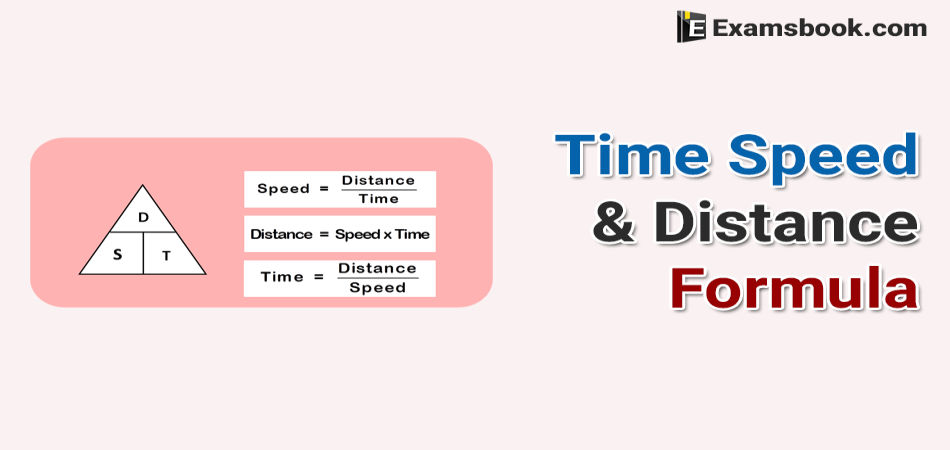Time, speed and distance is an important topic for competitive exams. Some of the students are facing problem while solving time, speed and distance questions in the exam. If you are facing the same problem in the competitive exam, you should know the correct way to use time, speed and distance formula.

Here given formulas will help you to save your time as well as increase your performance while solving Time Speed and Distance Questions in the exam. So, keep practice and learn how to use time speed and distance formula.

## Time Speed And Distance Formula

Time

Time is defined as quantity, which governs the order or sequence of an occurrence. In the absence of time, the actual sequence of any occurrence or incident would be lost. If we did not have the concept of time, we would not be able know in what period or in what order something took place.

Hence, Time =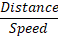Unit of Time: Hour (h) and second (s) are mostly taken unit of time.

Speed

Speed is defined as the distance covered per unit time. It is the rate at which the distance is covered.

Hence, Speed =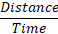Unit of Speed: Though we commonly take km/h. As units of speed.

Distance

When an object is moving with a certain speed in particular time, the displacement made by an object is called the distance.

Hence, Distance = Speed×Time

Unit of Distance: Kilometre (km) and metre (m) is usually taken as the unit of distance.

Keep In Mind

→ If two objects are in motion and their speeds are a and b respectively, then

● If two objects are moving in opposite direction, then relative speed = a+b.

● If two objects are moving in same direction, then relative speed = a – b

● a km/h =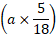m/s and b m/s =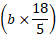km/h

● If the ratio of speed of A and B is x: y, then the ratio of time taken by them to cover same distance is given by 1/x: 1/y i.e. y: x.

Time Speed And Distance Formula With Example.

Example1.Shantanu covers a certain distance by car driving at 35 km/h and he returns back to starting point riding on a scooter with a speed of 25 km/h. Find the average speed for the whole journey.

(A) 30.15 km/h

(B) 29.16 km/h

(C) 28.16 km/h

(D) 30.02 km/h

When a certain distance is covered at speed A and the same distance is covered at speed B, then the average speed during the whole journey is given by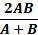Solution:

According to the formula,

Average speed = (2×35×25)/60     [ A = 35km/h, B = 25km/h]

= 70×25/60 = 175/6 = 29.16 km/h

Example2. Ashutosh covers a certain distance between his home and college by cycle. Having an average speed of 30 km/h, he is late by 20 min. However, with a speed of 40 km/h, he reaches his college 10 min earlier. Find the distance between his house and collage.

(A) 60 km

(B) 50 km

(C) 40 km

(D) 20 km

When a person covers a certain distance between two certain place with speed ‘a’, he gets his destination late by time t1 but when he covers the same distance with speed ‘b’. He reaches his destination t2 time earlier. In this case, the distance between two places is given by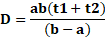Solution:

According to the formula, a = 30, b = 40

t1= 20/60, t2= 10/60

⸫ Required Distance =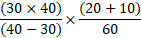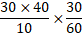= (30×4)×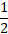= 60 km

Example3. A Motorcycle moves ate the speed of 80 km/hr. What is the speed of the car in meters per second?

(A) 8 m/sec

(B)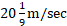(C) 15 m/sec

(D) None of these

km/hr to m/sec conversion

X km /hr =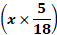m/sec.

Solution:

According to the formula,

Speed =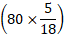m/sec = 200/9 m/sec  =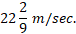Example4. An athlete runs 200 metres race in 24 second. His speed is:

(A) 20 km/hr

(B) 24 km/hr

(C) 28.5 km/hr

(D) 30 km/hr

m/sec to km/hr conversion

X m /sec =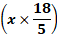km/hr.

Solution:

According to the formula,

Speed = (200/24) m/sec

= 25/3 m/sec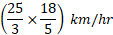= 30 km/hr.

Example5. Rajini’s can row 12 km/h in still water. It takes him twice as long to row up as to row down the river. Find the rate of stream.

(A) 3 km/h

(B) 5 km/h

(C) 4 km/h

(D) 6 km/h

If speed of stream is ‘a’ and a boat (or swimmer) takes ‘n’ times as long to row up as to row down the river, then speed of boat (or swimmer) in still water =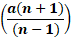Solution:

Given that,

Speed of Rajnish in still water = 12 km/h, n = 2

Speed of stream (a) = ?

According to the formula,

Speed in still water =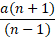→ 12 =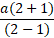→ 3a = 12

⸫ a = 12/3 = 4 km/h.

Example6. A, B and C walk 1 km in 5 min, 8 min and 10 min, respectively. C starts walking from a point, at a certain time, B starts from the same point 1 min later and A starts from the same point 2 min later than C. Then, A meet B and C at times

(A) 2 min, 3 min

(B) 4/3 min, 3 min

(C) 2  min, 5/3 min

(D) 1 min, 2 min

Solution:

A walks 1 km in 5 min.

Then, distance covered by A in 1 min = 1000/5 = 200 m

B walks 1 km in 8 min.

Then, distance covered by B in 1 min = 1000/8 = 125 m

C walks 1 km in 10 min.

Then, distance covered by C in 1 min = 1000/10 = 100 m

Let A meet B and C in x min and y min.

Then, according to the question,

Distance covered by C in (x+2) min

= Distance covered by A in x min

→ 100(x+2) = 200x

→ 100x + 200 = 200x

→ 200 = 100x

⸫  x = 200/100 = 2 min.

Now, for A and B

Distance covered by B in (y+1) min

= Distance covered by A in y min

→ 125(y+1) = 200 × y

→ 125 y + 125= 200y

→ 125 = 200y – 125 = 75 y

⸫ y = 125/75 = 5/3 min.

You can ask me anything related time speed and distance formula in the comment section if you facing any problem.